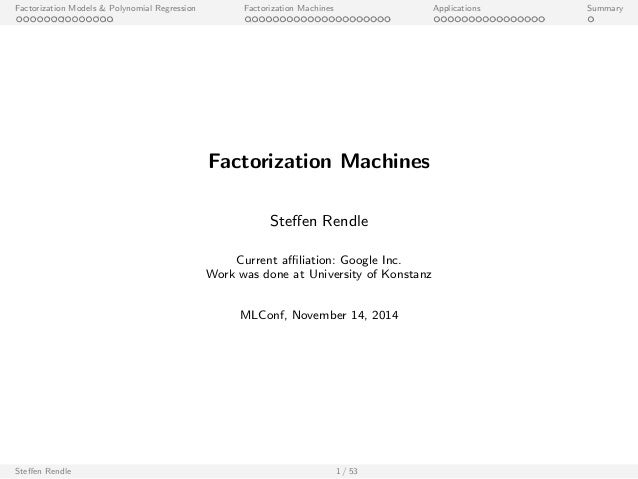Successfully reported this slideshow.
We use your LinkedIn profile and activity data to personalize ads and to show you more relevant ads. You can change your ad preferences anytime.Upcoming SlideShare
×

# Steffen Rendle, Research Scientist, Google at MLconf SF

5,697 views

Published on

Title: Factorization Machines

Abstract:
Developing accurate recommender systems for a specific problem setting seems to be a complicated and time-consuming task: models have to be defined, learning algorithms derived and implementations written. In this talk, I present the factorization machine (FM) model which is a generic factorization approach that allows to be adapted to problems by feature engineering. Efficient FM learning algorithms are discussed among them SGD, ALS/CD and MCMC inference including automatic hyperparameter selection. I will show on several tasks, including the Netflix prize and KDDCup 2012, that FMs are flexible and generate highly competitive accuracy. With FMs these results can be achieved by simple data preprocessing and without any tuning of regularization parameters or learning rates.

Published in: Technology
• Full Name
Comment goes here.

Are you sure you want to Yes No
Your message goes hereAre you sure you want to  Yes  No
Your message goes here

### Steffen Rendle, Research Scientist, Google at MLconf SF

1. 1. Factorization Models & Polynomial Regression Factorization Machines Applications Summary Factorization Machines Steen Rendle Current aliation: Google Inc. Work was done at University of Konstanz MLConf, November 14, 2014 Steen Rendle 1 / 53
2. 2. Factorization Models Polynomial Regression Factorization Machines Applications Summary Outline Factorization Models Polynomial Regression Factorization Models Linear/ Polynomial Regression Comparison Factorization Machines Applications Summary Steen Rendle 2 / 53
3. 3. Factorization Models Polynomial Regression Factorization Machines Applications Summary Matrix Factorization Example for data: Matrix Factorization: Movie TI NH SW ST ... 5 3 1 ? ... ? ? 4 5 ... 1 ? 5 ? ... ... ... ... ... ... A B C ... User ^ Y := W Ht ; W 2 RjUjk ;H 2 RjIjk k is the rank of the reconstruction. Steen Rendle 3 / 53
4. 4. Factorization Models Polynomial Regression Factorization Machines Applications Summary Matrix Factorization Example for data: Matrix Factorization: Movie TI NH SW ST ... 5 3 1 ? ... ? ? 4 5 ... 1 ? 5 ? ... ... ... ... ... ... A B C ... User ^ Y := W Ht ; W 2 RjUjk ;H 2 RjIjk ^y(u; i) = ^yu;i = Xk f =1 wu;f hi ;f = hwu; hi i k is the rank of the reconstruction. Steen Rendle 3 / 53
5. 5. Factorization Models Polynomial Regression Factorization Machines Applications Summary Matrix Factorization Extensions Example for data: Examples for models: Movie TI NH SW ST ... 5 3 1 ? ... ? ? 4 5 ... 1 ? 5 ? ... ... ... ... ... ... A B C ... User ^yMF(u; i ) := Xk f =1 vu;f vi ;f = hvu; vi i Steen Rendle 4 / 53
6. 6. Factorization Models Polynomial Regression Factorization Machines Applications Summary Matrix Factorization Extensions Example for data: Examples for models: Movie TI NH SW ST ... 5 3 1 ? ... ? ? 4 5 ... 1 ? 5 ? ... ... ... ... ... ... A B C ... User ^yMF(u; i ) := Xk f =1 vu;f vi ;f = hvu; vi i ^ySVD++(u; i) := * vu + X j2N(u) vj ; vi + ^yFact-KNN(u; i ) := 1 jR(u)j X j2R(u) ru;j hvi ; vj i Steen Rendle 4 / 53
7. 7. Factorization Models Polynomial Regression Factorization Machines Applications Summary Matrix Factorization Extensions Example for data: Examples for models: Movie TI NH SW ST ... 5 3 1 ? ... ? ? 4 5 ... 1 ? 5 ? ... ... ... ... ... ... A B C ... User ^yMF(u; i ) := Xk f =1 vu;f vi ;f = hvu; vi i ^ySVD++(u; i) := * vu + X j2N(u) vj ; vi + ^yFact-KNN(u; i ) := 1 jR(u)j X j2R(u) ru;j hvi ; vj i Rating Matrix time ^ytimeSVD(u; i ; t) := hvu + vu;t ; vi i ^ytimeTF(u; i ; t) := Xk f =1 vu;f vi ;f vt;f : : : Steen Rendle 4 / 53
8. 8. Factorization Models Polynomial Regression Factorization Machines Applications Summary Tensor Factorization Example for data: Examples for models: Triples of Subject, Predicate, Object ^yPARAFAC(s; p; o) := Xk f =1 vs;f vp;f vo;f ^yPITF(s; p; o) := hvs ; vpi + hvs ; voi + hvp; voi : : : Steen Rendle 5 / 53 [illustration from Drumond et al. 2012]
9. 9. Factorization Models Polynomial Regression Factorization Machines Applications Summary Sequential Factorization Models Example for data: Examples for models: Bt Bt­3 b b a b a c User 1 ? c e c c a ? d c e e ? ? User 2 User 3 User 4 Bt­2 Bt­1 a ^yFMC(u; i ; t) := X l2Bt